# Java Program to Make a Simple Calculator Using switch case

In this tutorial you will learn about the Java Program to Make a Simple Calculator Using switch case and its application with practical example.

In this tutorial, we will learn to create a Java program to make a simple Calculator using Switch case using Java programming.

## Prerequisites

Before starting with this tutorial we assume that you are best aware of the following Java programming topics:

• Java Operators.
• Basic Input and Output
• Class and Object.
• Basic Java programming.
• if-else statements.
• Switch case.
• Nested if-else Statements.
• Java Scanner class.

## What is calculator?

Calculator is a electronic device that is used to performing arithmetic operations on numbers.The Calculators  do  Addition, Subtraction,Multiplication, and Division… and function like that.

## Java Program to Make a Simple Calculator Using switch case

In this our program we will create a small calculator using switch case . We would first declared and initialized the required variables. Next, we would prompt user to input the two values . Later in the program we will ask a operator and values to perform that particular operation in our program.

## Output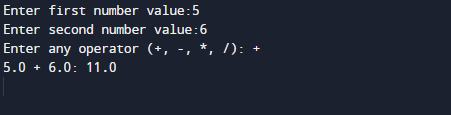In the above program, we have first declared and initialized a set variables required in the program.

• a= it will hold First value.
• b= it will hold second value.
• opt=it will hold operator value.

And  in the next statement user will be prompted to enter two values and  which will be assigned to variable ‘a‘ and ‘b‘ respectively.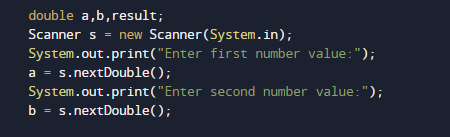And after taking the values form user we will ask to input symbol to perform following tasks.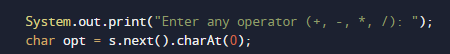After taking both the values of variable and opt we will pass the value of opt to switch case to Calculate values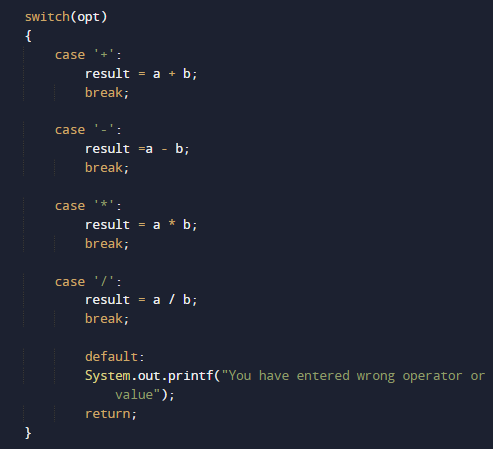The above  statements compute the addition of two numbers and print the output.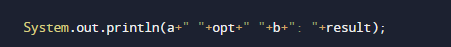Finally, with the break statement compiler ends the execution of program at switch statement.And we can perform different operation given different different operators and different values in our program as shown above in our program.

In this tutorial we have learn about the Java Program to Make a Simple Calculator Using switch case and its application with practical example. I hope you will like this tutorial.